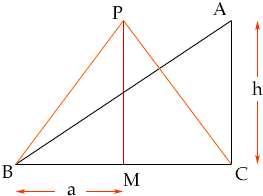Quandaries and Queries I am a student (10-12) secondary. Please could you tell me why for my coursework (where I have to find the largest area that a fence 1000m long can cover) why I should only test equilateral and isoceles triangles? We were told NOT to do right angled triangles but I was wondering why not? Thankyou very much Petey Hi Petey Suppose that triangle ABC has a right angle at C and that the perimeter is 1000 m. Let M be the midpoint of BC. Construct an isosceles triangle PBC on the same base, BC, and with |CA| = |PM|. The triangles ABC and PBC have the same base and height and hence the same area. I want to show you that the perimeter of triangle PBC is less than the perimeter of triangle ABC.Claim: |PB| + |CP| = 2 |PB| < |AB| + |CA|. Since all the lengths are positive it is sufficient to show that 4 |PB|2 < (|AB| + |CA|)2 Let h = |AC| = |PM| and a = |BM| = |MC|. Using Pythagoras' Theorem, |PB|2 = a2 + h2 and |AB|2 = 4 a2 + h2 Thus (|AB| + |CA|)2 = |AB|2 + |CA|2 + 2 |AB||CA| = 4 a2 + h2 + h2 + 2 h Sqrt( 4 a2 + h2) > 4 a2 + 2 h2 + 2 h Sqrt(h2) = 4 a2 + 4 h2 = 4 |PB|2 Thus the perimeter of triangle PBC is less than the perimeter of triangle ABC. Hence if you move P upwards to the place where the triangles have the same perimeter you have a new isoscelese triangle PBC with the same primeter as ABC but a larger area. Cheers, Penny Go to Math Central